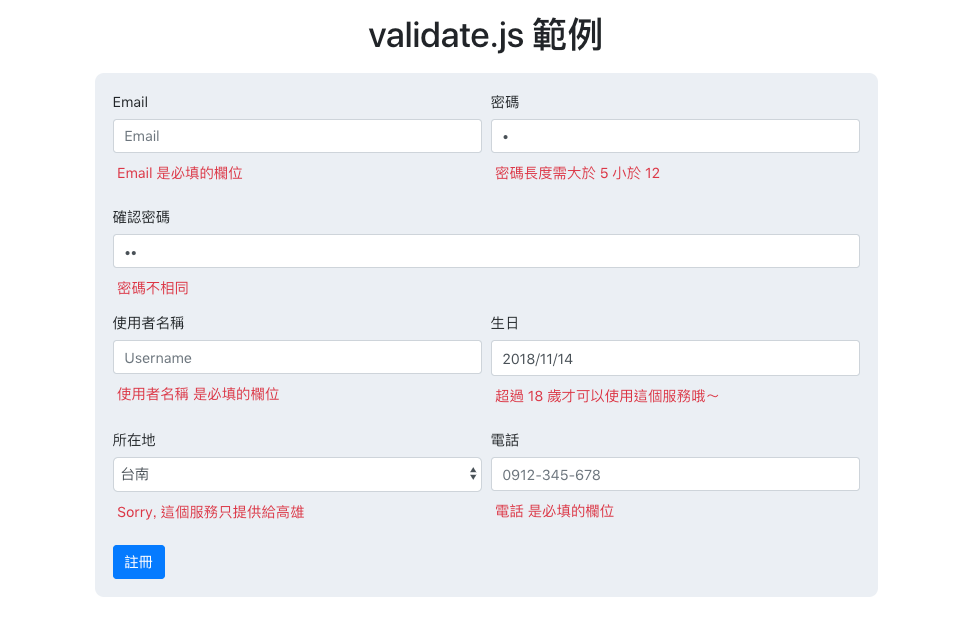#2019 iT 邦幫忙鐵人賽DAY 7
2
Modern Web

## validate.js - 輕鬆完成表單驗證

validate.js 是一個方便用於表單驗證的 JavaScript 函式庫，透過 validate.js 你可以更簡單且完整的處理表單驗證的問題，那就來介紹吧！

## 安裝

### 瀏覽器 / CDN

``````<script src="//cdnjs.cloudflare.com/ajax/libs/validate.js/0.12.0/validate.min.js"></script>
``````

### npm / node.js

``````\$ npm install --save validate.js
``````
``````var validate = require("validate.js");
``````

## 格式

``````<attribute>: {
<validator name>: <validator options>
}
``````

``````email: {
presence: true, // Email 是必填欄位
email: true // 需要符合 Email 格式
},
``````

### attribute

`<attribute>` 的部分會對應 input 的 name 來設置，舉例：

``````<input id="email" class="form-control" type="email" placeholder="Email" name="email">
``````

### validator name

``````presence: true
``````

#### datetime

``````"birthDate": {
datetime: {
dateOnly: true,
latest: moment.utc().subtract(18, 'years'),
message: "^You need to be atleast 18 years old"
}
}
``````

#### Email

``````"Email": {
email: true,
}
``````

#### Equality

``````"confirm-password": {
equality: {
message: "^The passwords does not match",
}
}
``````

#### Exclusion

``````"country": {
exclusion: {
within: ["SE"],
message: "^Sorry, this service is for Sweden only",
}
},
``````

#### Inclusion

``````"country": {
inclusion: {
within: ["SE"],
message: "^Sorry, this service is for Sweden only",
}
},
``````

#### Format

• g：全域比對
• i：忽略大小寫
``````"username": {
format: {
pattern: "[a-z0-9]+", // 只能包含數字或英文字
flags: "i", // 忽略大小寫
message: "can only contain a-z and 0-9",
}
},
``````

#### Length

``````"password": {
length: {
minimum: 5, // 輸入值不能短於此值
maximum: 20, // 輸入值不能長於此值
}
},
``````

#### Numericality

``````numericality: {
onlyInteger: true, // 只能是整數
greaterThanOrEqualTo: 0, // 只能大於等於零
}
``````

#### URL

``````"website": {
url: {
allowLocal: true,
}
}
``````

## Utilities

### contains

``````validate.contains(collection, value)
``````

``````validate.contains({}, "foo");// => false
validate.contains({foo: "bar"}, "foo");// => true
validate.contains([1, 2, 3], 4);// => false
validate.contains([1, 2, 3], 3);// => true
``````

### isBoolean

``````validate.isBoolean(value)
``````

``````validate.isBoolean("true");// => false
validate.isBoolean(true);// => true
``````

### isDate

``````validate.isDate(value)
``````

``````validate.isDate(new Date());// => true
validate.isDate(null);// => false
validate.isDate({});// => false
``````

### isEmpty

``````validate.isEmpty(value)
``````

``````validate.isEmpty({});// => true
validate.isEmpty(null);// => true
validate.isEmpty("");// => true
validate.isEmpty("   ");// => true
validate.isEmpty("foo");// => false
validate.isEmpty({foo: "bar"});// => false
``````

## 驗證功能

### Single value validation：單值驗證

``````validate.single("foo", {presence: true, email: true});
// => ["is not a valid email"]
``````

### Nested validation：巢狀驗證

``````var constraints = {
presence: true
},
format: {
// Must be numbers followed by a name
pattern: "^[0-9]+ .+\$",
message: "^The street for the shipping address must be a valid street name"
}
}
};

validate({}, constraints);
``````